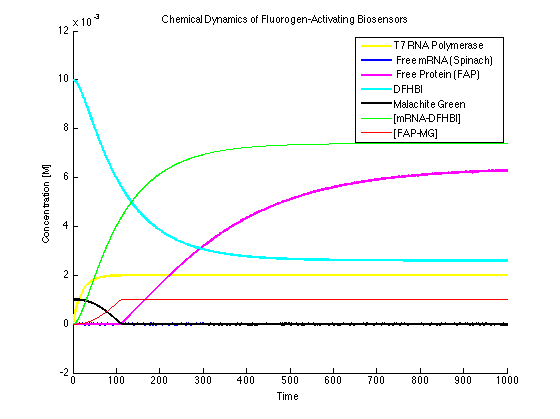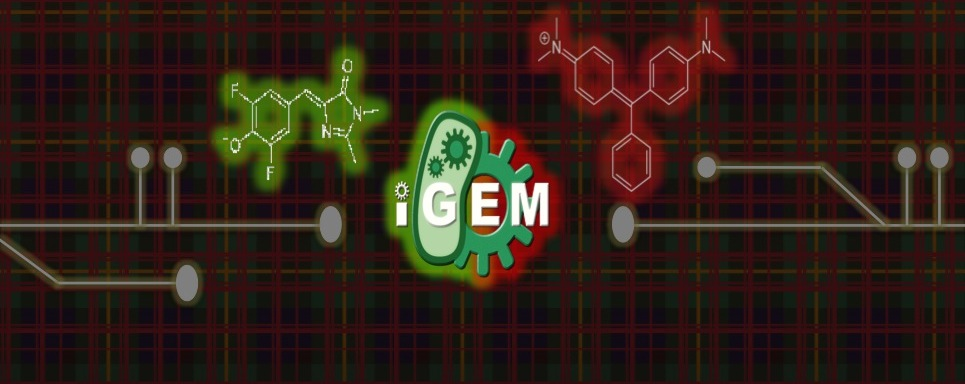# Team:Carnegie Mellon/Mod-Expanded

(Difference between revisions)
 Revision as of 03:55, 27 October 2012 (view source)Ychoo (Talk | contribs)← Older edit Revision as of 03:55, 27 October 2012 (view source)Ychoo (Talk | contribs) Newer edit → Line 152: Line 152:

- Figure 1
+ Figure 1 The quantities circled in red are measurable or approximated in our model. The only two physical quantities not known are the RNA and protein concentrations. These two can be solved by using a fitting function of the simulation. - + - The quantities circled in red are measurable or approximated in our model. The only two physical quantities not known are the RNA and protein concentrations. These two can be solved by using a fitting function of the simulation. +

## Revision as of 03:55, 27 October 2012# Overview

Our original model was based on the assumption that the T7 RNA polymerase is constitutively expressed and thus that IPTG addition instantaneously “turns on” the expression of the mRNA and FAP (reactions 3-12 in Figure 1). In this model, reactions 3 - 7 involve mRNA. The T7 RNAP polymerase is not consumed in producing mRNA and mRNA is not consumed in producing the FAP.

We assumed that the fluorescence signal is proportional to the concentration of the [mRNA-DFHBI]. The proportionality constant K includes factors specific to the fluorimeter settings and K was one of the variable parameters in our fitting algorithm.

The dosage curve indicated that the dye binding reactions (6, 7 and 10, 11) are not rate-limiting and allowed us to measure the equilibrium constant KD for these reactions. KD is equal to the ratio of the “off” rate (kd) to “on” rate (ka).

The degradation of [dye-mRNA] and [dye-FAP] complexes affects only the biological component of the complex and not the dye. We assumed that the two complexes degrade with the same rate constant, α and β, as their mRNA and FAP unbound counterparts, respectively.

The model described above could not lead to good simulations of the time dependence of the fluorescence of the [mRNA-DFHBI] complex. The apparent exponential dependence of the [mRNA-DFHBI] on time could be rationalized if one includes in the model two additional reaction that account for the fact that in the regulatory mechanism the T7 RNA polymerase in the BL21(DE3) cell strain, there is a LacO site upstream of the promoter for the polymerase. We accounted for this process by including reactions 1 and 2 in the Figure 1. Reaction 1 represents the induction of the T7 RNAP by adding a fixed amount of IPTG and reaction 2 represents the degradation of T7 RNAP.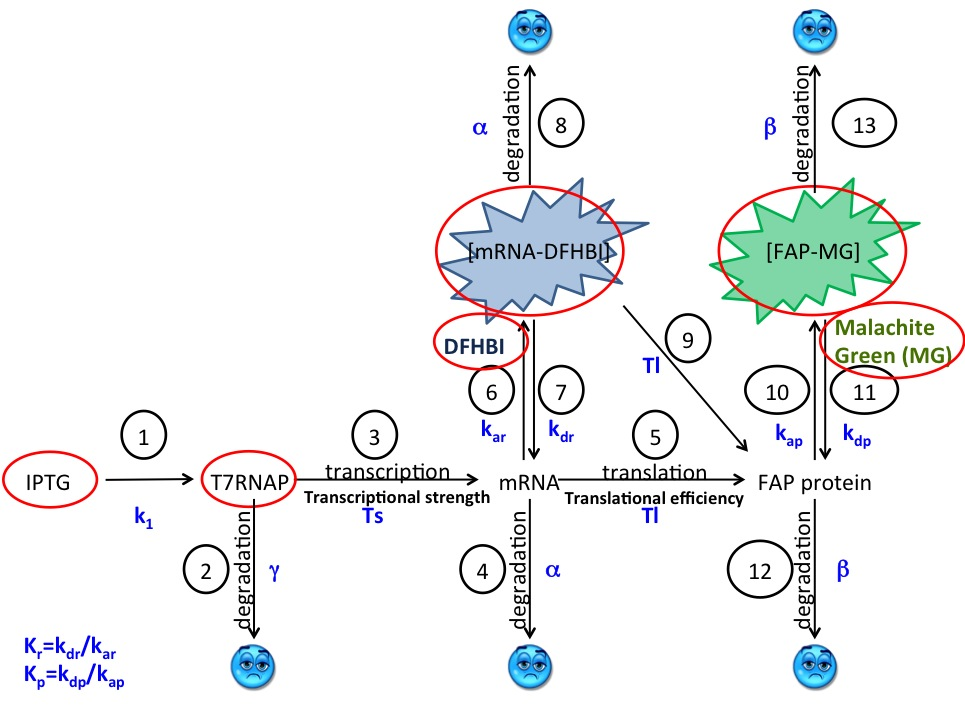Figure 1 The quantities circled in red are measurable or approximated in our model. The only two physical quantities not known are the RNA and protein concentrations. These two can be solved by using a fitting function of the simulation.

The following system of differential equations represent the chemical reactions in Figure 1.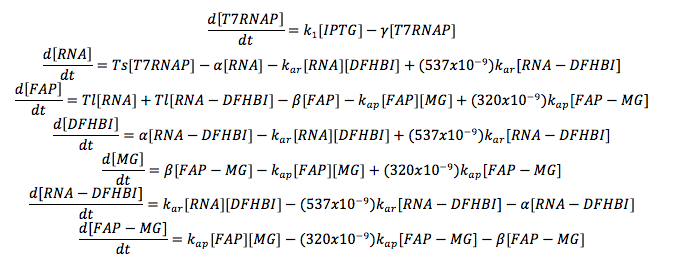Figure 2 KD's were taken from the original papers Szent-Gyorgi et al. and Paige et al.

The initial concentrations of dyes and IPTG are known. The [IPTG] value is constant. k1 should be determined so that the steady state for T7RNAP is accurate. We implemented these equations in MATLAB using an ODE function and a stochastic simulation approach.

For low dye concentration, the fluorescence increases until all the dye is bound. One can take advantage of this property in a titration experiment to determine the maximum concentration of RNA or protein produced by the cell.

All dye bound condition (Figure 3):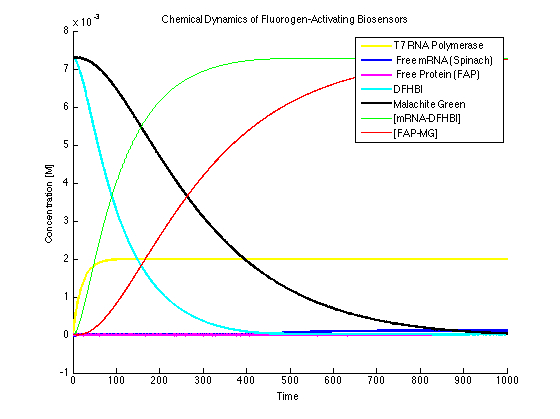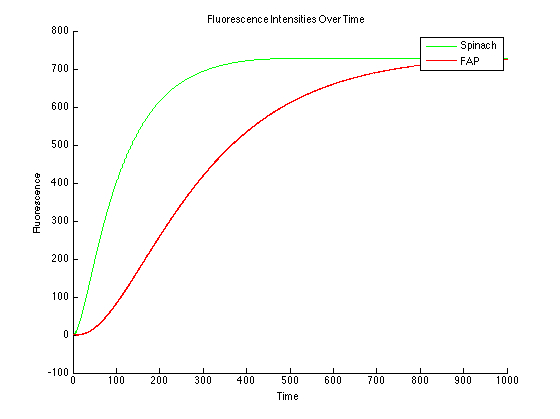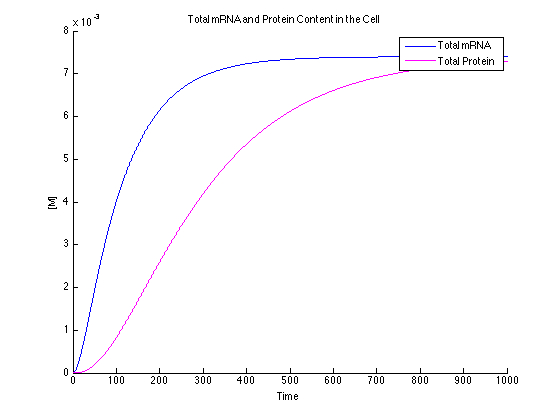It can be seen that the MG and DFHBI have been added in an amount to minimize the amount of free protein and RNA. This value can be determined experimentally.

Excess dye condition (Figure 4):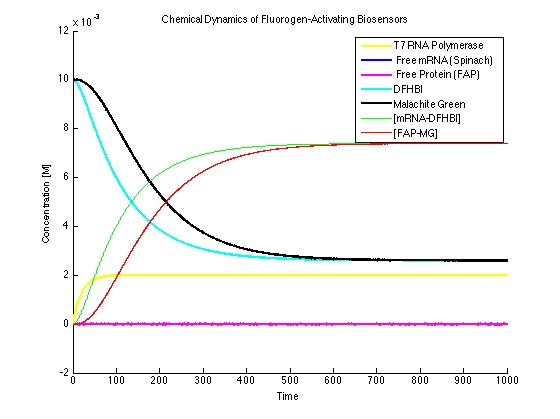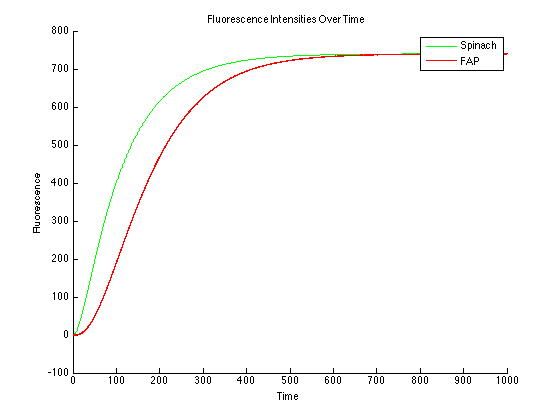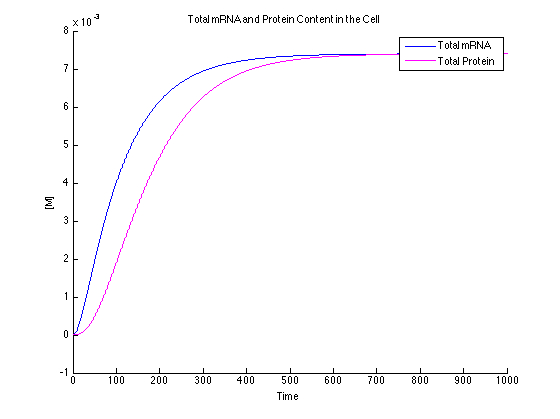In this case, the dyes are in excess. This will cause the data to be too high and should be avoided experimentally.